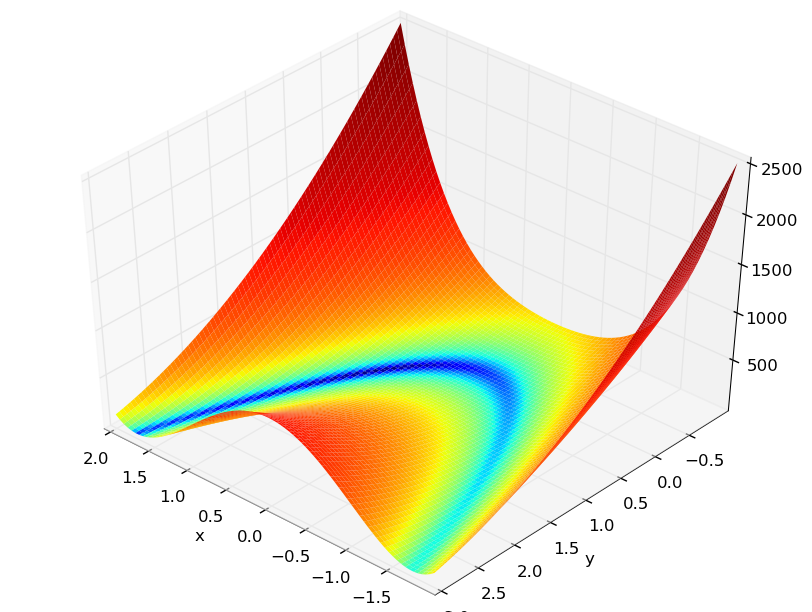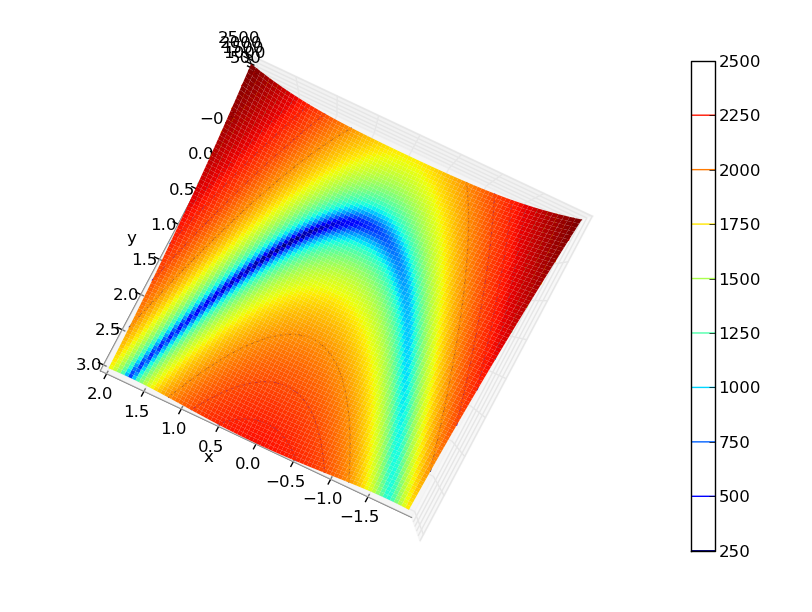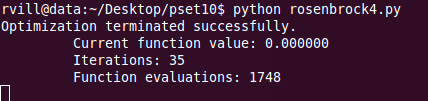The Nature of Mathematical Modeling - Rachelle Villalon

Search

 Plot the Rosenbrock function:<Although Mathematica can plot the rosenbrock function with just one line of code, using Matplotlib in Python appears to offer more graphical output options if you're patient enough to write a few extra lines of code. Here it is in Mathematica: rosenbrock.nb. Working this problem in python, the results from evaluating the function using the downhill simplex method can be seen in the screenshot below:Similarly, after evaluating the function using the direction set method I obtain the following results:My implementation of the results above: rosenbrock4.py. This week has been a balancing act between diving into my final project (the first part of the agenda: understanding Navier-Stokes equations and basic fluid dynamics algorithms) and studying this week's topic. The following implementation is a work in progress - I'll need to fix some issues that I've come across when computing the conjugate gradient and Levenberg-Marquardt method. .textContainer { font-family: "HelveticaNeue-Light", "Helvetica Neue Light", "Helvetica Neue", Helvetica, Arial, "Lucida Grande", sans-serif; font-weight: 300; font-size: 15px; }